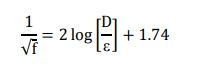# How to find Head Loss or Friction Loss or Frictional Pressure Drop ?

Definition :-
Head Loss is the measure of the reduction in the total head of the liquid as it moves through a pipe. The total head is the sum of the elevation head (height), velocity head and pressure head. Head loss is unavoidable and is present because of the friction between the fluid and the walls of the pipe and is also present between adjacent fluid particles as they flow along the pipe.

Overall head loss in a pipe is affected by a number of factors which include the viscosity of the fluid, the size of the internal pipe diameter, the internal roughness of the inner surface of the pipe, the change in elevation between the ends of the pipe and the length of the pipe along which the fluid travels.

Head loss is the combination of different types of losses :-
1.Friction Losses ,
2.Minor Losses

Friction Losses are head losses that occur due to friction from the walls of the pipe on the liquid. Friction losses are very dependent upon the viscosity of the liquid and the amount of turbulence in the flow.
Minor Losses are head losses that occur due to bends, Elbows, Joints, Valves, and other fittings in the systems. Whenever there is a change in the direction of flow or a change in the cross-sectional area a head loss will occur. Minor losses are usually negligible compared to friction losses in larger pipe systems.

Typical roughness values are mentioned in below table for different pipe materials :-

Head losses through a straight pipe or Friction loss :-

Darcy-Weisbach equation :-
Pressure loss in steady pipe flow is calculated using the Darcy-Weisbach equation. This equation includes the Darcy friction factor. The exact solution of the Darcy friction factor in turbulent got by looking at the Moody diagram or by solving it from the Colebrook equation or solving from some explicit approximations like chen, barr, swamee & jain etc.

The head loss hf due to friction undergone by a fluid motion in a pipe is usually calculated through the Darcy-Weisbach relation as :-

f = Darcy friction factor
L = Characteristics length of the pipe
D = Diameter of the pipe,
V = Velocity of the flow of liquid
g = Acceleration due to the gravity.

Calculation of Friction factor :-
Before the pipe losses can be estimated, the friction factor must be calculated. The friction factor will be dependent on the pipe size, inner roughness of the pipe, flow velocity and fluid viscosity. The flow condition, whether „turbulent‟ or not, will be the method used to calculate the friction factor. There are many equations used for calculation of friction factor. Some of them namely, Chen approximation, Barr approximation, Swamee and Jain approximation, Zigrang and Sylvester approximation and Romeo, Royo and Monzon approximation are used in this particular study.

Hagen-Poiseuille Equation :-
Hagen-Poiseuille proposed an equation which is widely used for calculation of friction factor.
We can just equate the Hagen-Poiseuille and the Darcy-Weisbach Equations:

Hence, for laminar flow we have:

The friction factor is linearly dependent on R,

Where, R, the Reynolds number. Whereas, in turbulent flow (R ≥ 2000), the friction factor, f depends upon the Reynolds number (R) and on the relative roughness of the pipe, ε/D. The general behavior of turbulent pipe flow in the presence of surface roughness is well established. When € is very small compared to the pipe diameter D i.e. ε/D→0 When € is very small compared to the pipe diameter D i.e. ε/D→0, f depends only on R. When ε/D is of a significant value, at low R, the flow can be considered as in smooth regime (there is no effect of roughness). As R increases, the flow becomes transitionally rough, called as transition regime in which the friction factor rises above the smooth value and is a function of both € and R and as R increases more and more, the flow eventually reaches a fully rough regime in which f is independent of R.

In a smooth pipe flow, the viscous sub layer completely submerges the effect of roughness on the flow. In this case, the friction factor f is a function of R and is independent of the effect of roughness on the flow.

Blasius Equation :-
Blasius determined an equation from experiments on „smooth‟ pipes. The Blasius equation is the most simple equation for solving the Darcy friction factor because the Blasius equation has no term for pipe roughness, is valid only to smooth pipes. It is widely used for calculation of friction factor of turbulent flow. The Blasius equation is valid only for smooth pipe.

Prandtl equation :-
Prandtl equation was widely used for turbulent regime in smooth pipes which is implicit in friction factor. Prandtl derived a formula from the logarithmic velocity profiles and experimental data on smooth pipes.